### Home > MC1 > Chapter 6 > Lesson 6.2.5 > Problem6-94

6-94.
1. . For each of the following products, estimate approximately how big the answer should be. Explain your reasoning. Then multiply each set of numbers to see how close you were. Homework Help ✎

1.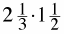2.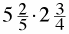3.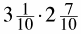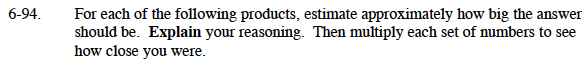To estimate the product of each expression in parts (a), (b), and (c),
try rounding the mixed numbers to the nearest whole number, then multiply.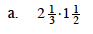To calculate the actual value of this expression, it would be best to convert each mixed number to a fraction greater than one. For instance, there are 3 thirds in 1, and 6 thirds in 2.

$\text{This means }2\frac{1}{3}\text{ is equal to }\frac{7}{3}.$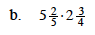Try making the mixed number into a decimal before multiplying.

$\text{The product of this expression is }14\frac{17}{20}$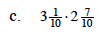A good estimate for this would be (3)(3).

The product of this expression is 8.37.

Refer to the Math Notes box in Lesson 6.2.6 for help with multiplying mixed numbers.